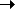Package net.flashpunk.graphics Class public class Emitter Inheritance EmitterGraphicObject

Particle emitter used for emitting and rendering particle sprites. Good rendering performance with large amounts of particles.

Public Properties
PropertyDefined Byactive : Boolean = false
If the graphic should update.
Graphic
particleCount : uint
[read-only] Amount of currently existing particles.
Emitterrelative : Boolean = true
If the graphic should render at its position relative to its parent Entity's position.
GraphicscrollX : Number = 1
X scrollfactor, effects how much the camera offsets the drawn graphic.
GraphicscrollY : Number = 1
Y scrollfactor, effects how much the camera offsets the drawn graphic.
Graphicvisible : Boolean = true
If the graphic should render.
Graphicx : Number = 0
X offset.
Graphicy : Number = 0
Y offset.
Graphic
Protected Properties
PropertyDefined Byassign : Function
Graphic
Public Methods
MethodDefined By

Emitter(source:*, frameWidth:uint = 0, frameHeight:uint = 0)
Constructor.
Emitter

emit(name:String, x:Number, y:Number):Particle
Emits a particle.
Emitter

newType(name:String, frames:Array = null):ParticleType
Creates a new Particle type for this Emitter.
Emitterrender(target:BitmapData, point:Point, camera:Point):void
Renders the graphic to the screen buffer.
Graphic

setAlpha(name:String, start:Number = 1, finish:Number = 0, ease:Function = null):ParticleType
Sets the alpha range of the particle type.
Emitter

setColor(name:String, start:uint = 0xFFFFFF, finish:uint = 0, ease:Function = null):ParticleType
Sets the color range of the particle type.
Emitter

setGravity(name:String, gravity:Number = 0, gravityRange:Number = 0):ParticleType
Sets the gravity range for a particle type.
Emitter

setMotion(name:String, angle:Number, distance:Number, duration:Number, angleRange:Number = 0, distanceRange:Number = 0, durationRange:Number = 0, ease:Function = null):ParticleType
Defines the motion range for a particle type.
Emitter

setRotation(name:String, startAngle:Number, spanAngle:Number, startAngleRange:Number = 0, spanAngleRange:Number = 0, smooth:Boolean = false, ease:Function = null):ParticleType
Defines the rotation range for a particle type.
Emitter

setSource(source:*, frameWidth:uint = 0, frameHeight:uint = 0):void
Changes the source image to use for newly added particle types.
Emitter

update():void
[override]
Emitter
Property Detail
 particleCount property
`particleCount:uint`  [read-only]

Amount of currently existing particles.

Implementation
`    public function get particleCount():uint`
Constructor Detail
 Emitter () Constructor
`public function Emitter(source:*, frameWidth:uint = 0, frameHeight:uint = 0)`

Constructor. Sets the source image to use for newly added particle types.

Parameters
 `source:*` — Source image. `frameWidth:uint` (default = `0`) — Frame width. `frameHeight:uint` (default = `0`) — Frame height.
Method Detail
 emit () method
` public function emit(name:String, x:Number, y:Number):Particle`

Emits a particle.

Parameters

 `name:String` — Particle type to emit. `x:Number` — X point to emit from. `y:Number` — Y point to emit from.

Returns
 `Particle` —
 newType () method
` public function newType(name:String, frames:Array = null):ParticleType`

Creates a new Particle type for this Emitter.

Parameters

 `name:String` — Name of the particle type. `frames:Array` (default = `null`) — Array of frame indices for the particles to animate.

Returns
 `ParticleType` — A new ParticleType object.
 setAlpha () method
` public function setAlpha(name:String, start:Number = 1, finish:Number = 0, ease:Function = null):ParticleType`

Sets the alpha range of the particle type.

Parameters

 `name:String` — The particle type. `start:Number` (default = `1`) — The starting alpha. `finish:Number` (default = `0`) — The finish alpha. `ease:Function` (default = `null`) — Optional easer function.

Returns
 `ParticleType` — This ParticleType object.
 setColor () method
` public function setColor(name:String, start:uint = 0xFFFFFF, finish:uint = 0, ease:Function = null):ParticleType`

Sets the color range of the particle type.

Parameters

 `name:String` — The particle type. `start:uint` (default = `0xFFFFFF`) — The starting color. `finish:uint` (default = `0`) — The finish color. `ease:Function` (default = `null`) — Optional easer function.

Returns
 `ParticleType` — This ParticleType object.
 setGravity () method
` public function setGravity(name:String, gravity:Number = 0, gravityRange:Number = 0):ParticleType`

Sets the gravity range for a particle type.

Parameters

 `name:String` — The particle type. `gravity:Number` (default = `0`) — Gravity amount to affect to the particle y velocity. `gravityRange:Number` (default = `0`) — Random amount to add to the particle's gravity.

Returns
 `ParticleType` — This ParticleType object.
 setMotion () method
` public function setMotion(name:String, angle:Number, distance:Number, duration:Number, angleRange:Number = 0, distanceRange:Number = 0, durationRange:Number = 0, ease:Function = null):ParticleType`

Defines the motion range for a particle type.

Parameters

 `name:String` — The particle type. `angle:Number` — Launch Direction. `distance:Number` — Distance to travel. `duration:Number` — Particle duration. `angleRange:Number` (default = `0`) — Random amount to add to the particle's direction. `distanceRange:Number` (default = `0`) — Random amount to add to the particle's distance. `durationRange:Number` (default = `0`) — Random amount to add to the particle's duration. `ease:Function` (default = `null`) — Optional easer function.

Returns
 `ParticleType` — This ParticleType object.
 setRotation () method
` public function setRotation(name:String, startAngle:Number, spanAngle:Number, startAngleRange:Number = 0, spanAngleRange:Number = 0, smooth:Boolean = false, ease:Function = null):ParticleType`

Defines the rotation range for a particle type.

Parameters

 `name:String` — The particle type. `startAngle:Number` — Starting angle. `spanAngle:Number` — Total amount of degrees to rotate. `startAngleRange:Number` (default = `0`) — Random amount to add to the particle's starting angle. `spanAngleRange:Number` (default = `0`) — Random amount to add to the particle's span angle. `smooth:Boolean` (default = `false`) — Whether to smooth the resulting rotated particle. `ease:Function` (default = `null`) — Optional easer function.

Returns
 `ParticleType` — This ParticleType object.
 setSource () method
` public function setSource(source:*, frameWidth:uint = 0, frameHeight:uint = 0):void`

Changes the source image to use for newly added particle types.

Parameters

 `source:*` — Source image. `frameWidth:uint` (default = `0`) — Frame width. `frameHeight:uint` (default = `0`) — Frame height.

 update () method
`override public function update():void`